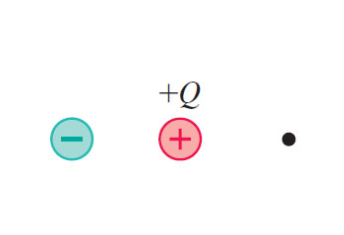# Problem: The positive charge in (Figure 1) is +Q.What is the negative charge if the electric field at the dot is zero?

###### FREE Expert Solution

Electric field:

$\overline{){\mathbf{E}}{\mathbf{=}}\frac{\mathbf{k}\mathbf{Q}}{{\mathbf{r}}^{\mathbf{2}}}}$

The field will be in the opposite direction because of the negative charge.

For the electric field to be zero, the magnitude of the field must be equal to the field due to Q.

91% (241 ratings)###### Problem Details

The positive charge in (Figure 1) is +Q.

What is the negative charge if the electric field at the dot is zero?